5.4.5 Symmetric Case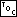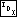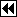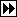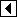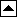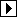Finally, we need to write code for the case that we chose not to discuss earlier, where the insertion occurs in the right subtree of y. All we have to do is invert the signs of balance factors and switch avl_link[] indexes between 0 and 1. The results are this:

```157. <Rebalance AVL tree after insertion in right subtree 157> =
struct avl_node *x = y->avl_link;
if (x->avl_balance == +1)
{     <Rotate left at y in AVL tree 158>   }
else   {     <Rotate right at x then left at y in AVL tree 159>   }
```

This code is included in 151 and 162.

```158. <Rotate left at y in AVL tree 158> =
w = x;
x->avl_balance = y->avl_balance = 0;
```

This code is included in 157 and 532.

```159. <Rotate right at x then left at y in AVL tree 159> =
assert (x->avl_balance == -1);